# Gauss interpolation formula

A formula in which the nodes (cf. Node) nearest to the interpolation pointare used as interpolation nodes. If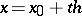, the formula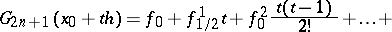(1)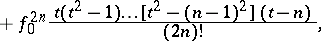written with respect to the nodes,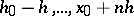,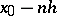is called the Gauss forward interpolation formula, while the formula(2)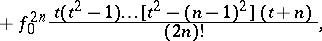written with respect to the nodes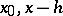,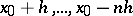,is called the Gauss backward interpolation formula, , . Formulas (1) and (2) employ finite differences, defined as follows: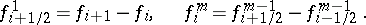The advantage of Gauss' interpolation formulas consists in the fact that this selection of interpolation nodes ensures the best approximation of the residual term of all possible choices, while the ordering of the nodes by their distances from the interpolation point reduces the numerical error in the interpolation.